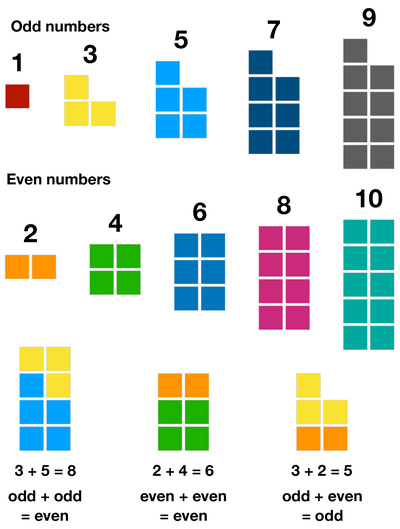# Number Blocks: Odd and Even numbersNatural numbers are either even or odd. An even number can be divided by 2 with no remainders left (or with a remainder of 0). Even numbers end in 2, 4, 6, 8, and 0. An odd number is not divisible evenly by 2, and its remainder is 1. Odd numbers end in 1, 3, 5, 7, and 9. Odd numbers are in between even numbers.

Differentiating even and odd numbers is a very important skill for kids, because it lays the foundation for learning mathematical concepts, such as division, prime numbers and even square roots. Kids should be confident with this skill when they are in the first or second grade.

This worksheet provides the first odd and even numbers up to 10. With the illustration, kids could easily grasp the concept of odd and even numbers: even numbers can be divided into two equal groups with no leftovers, while odd numbers cannot be divided into two equal groups without leftovers. It also shows the basic property of additions of odd and even numbers: Odd plus odd is even, even plus even is even, and odd plus even is odd. This is a very effective and efficient tool to teach kids how to differentiate odd and even numbers.